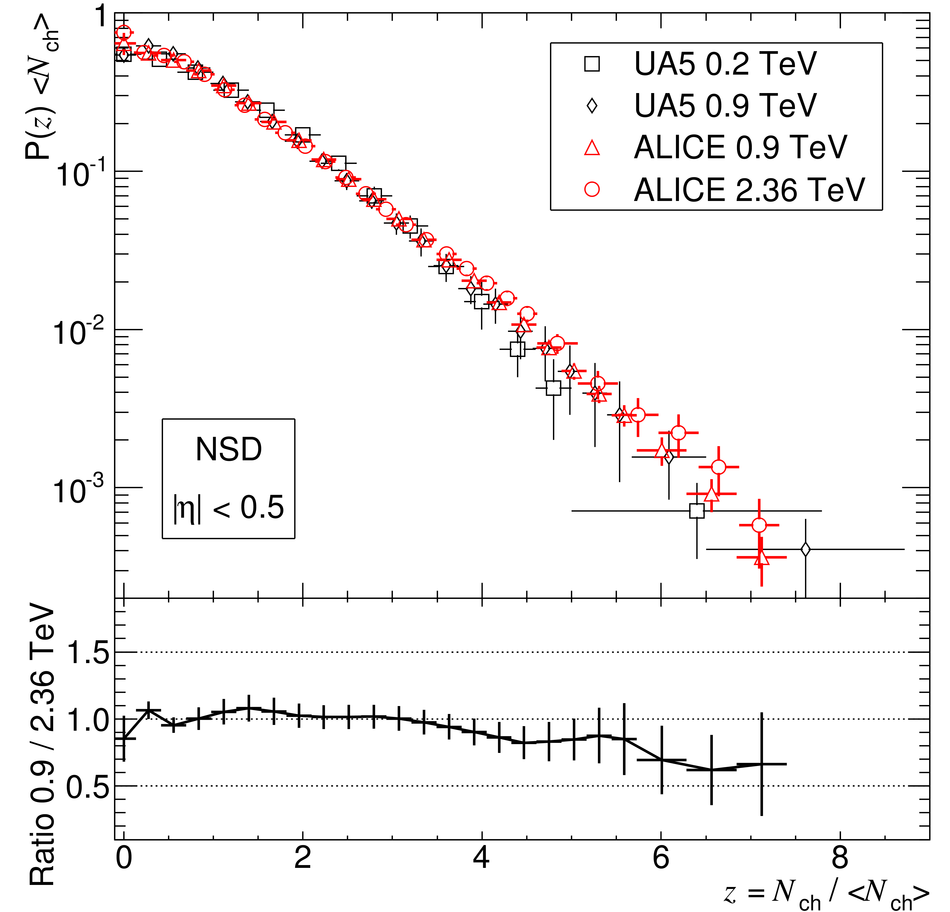# Charged-particle multiplicity measurement in proton-proton collisions at $\sqrt{s}$ = 0.9 and 2.36 TeV with ALICE at LHC

Charged-particle production was studied in proton-proton collisions collected at the LHC with the ALICE detector at centre-of-mass energies 0.9 TeV and 2.36 TeV in the pseudorapidity range |$\eta$| < 1.4. In the central region (|$\eta$| < 0.5), at 0.9 TeV, we measure charged-particle pseudorapidity density ${\rm d}N_{\rm ch}/{\rm d}\eta$ = 3.02 +- 0.01 (stat.) +0.08 -0.05 (syst.) for inelastic interactions, and ${\rm d}N_{\rm ch}/{\rm d}\eta$ = 3.58 +- 0.01 (stat.) +0.12 -0.12 (syst.) for non-single-diffractive interactions. At 2.36 TeV, we find ${\rm d}N_{\rm ch}/{\rm d}\eta$ = 3.77 +- 0.01 (stat.) +0.25 -0.12 (syst.) for inelastic, and${\rm d}N_{\rm ch}/{\rm d}\eta$ = 4.43 +- 0.01 (stat.) +0.17 -0.12 (syst.) for non-single-diffractive collisions. The relative increase in charged-particle multiplicity from the lower to higher energy is 24.7% +- 0.5% (stat.) +5.7% -2.8% (syst.) for inelastic and 23.7% +- 0.5% (stat.) +4.6% -1.1% (syst.) for non-single-diffractive interactions. This increase is consistent with that reported by the CMS collaboration for non-single-diffractive events and larger than that found by a number of commonly used models. The multiplicity distribution was measured in different pseudorapidity intervals and studied in terms of KNO variables at both energies. The results are compared to proton-antiproton data and to model predictions.

Figures

## Figure 1

 Distributions of reconstructed event vertices along the beam direction ($z$) obtained from hit correlations in the two pixel layers of the ALICE inner tracking system for the event samples used in the analysis (see text): $\sqrt{s}$ = 0.9 TeV (full symbols) and $\sqrt{s}$ = 2.36] TeV (open symbols). The lines are from Monte Carlo simulations Vertical dashed lines delimit the region $|z|< 10~$cm, where the events for the present analysis were selected.## Figure 2

 Charged-particle multiplicity dependence of the selection efficiency, for INEL events (triangles) with MB$_{\mathrm{OR}}$ selection, and for NSD (circles) and SD (squares) events with MB$_{\mathrm{AND}}$ selection obtained with a simulation of the $0.9~$TeV data.## Figure 3

 Measured raw multiplicity distributions in three pseudorapidity ranges for both energy samples ($0.9~$TeV full lines, $2.36~$TeV dashed lines). Note that for |$\eta$| < 1.0 and |$\eta$|< 1.3 the distributions have been scaled for clarity by the factor indicated.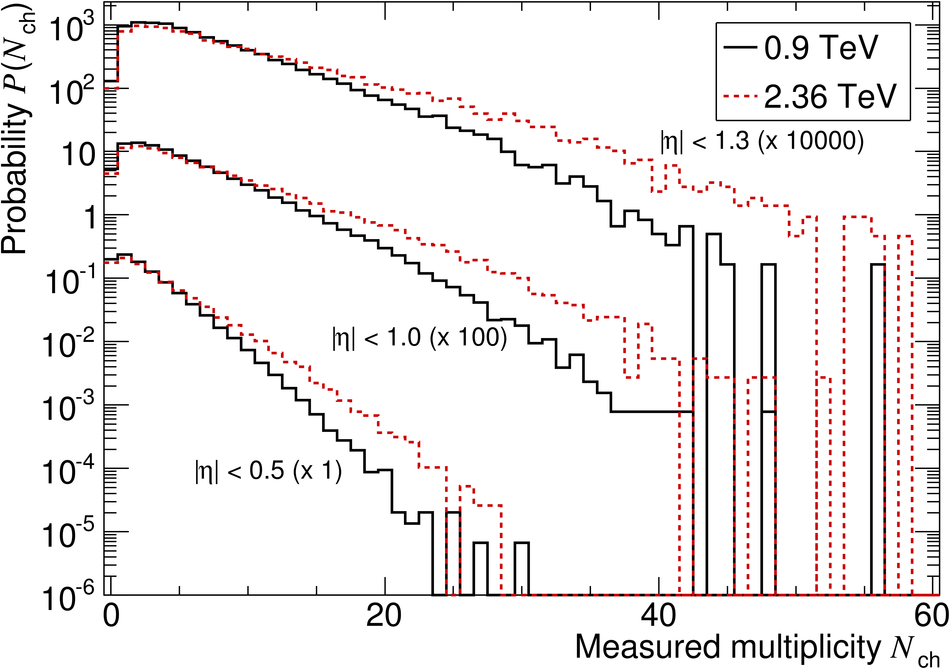## Figure 4

 Graphical representation of the detector response matrix: number of tracklets found in the SPD ($m$) vs the number of generated primary particles in $|\eta| < 1.0$ ($t$) for $\sqrt{s} = 0.9~$TeV The distribution of the measured tracklet multiplicity for a given generated multiplicity shown with its most probable value (dots), r.m.s. (shaded areas), and full spread (squares).## Figure 5

 Measured raw multiplicity distribution (elements of vector $M$, histogram), superimposed on the convolution $RU$ of the unfolded distribution with the response matrix (crosses), at $\sqrt{s} = 0.9~$TeV for $|\eta| < 1.0$ (upper plot). The error bars are omitted for visibility Normalized residuals, i.e. the difference between the measured raw distribution and the corrected distribution folded with the response matrix divided by the measurement error (lower plot) The inset shows the distribution of these normalized residuals fitted with a Gaussian ($\sigma \approx 1.06$).## Figure 6

 Top: Measured pseudorapidity dependence of $\dNdEta$ at $\sqrt{s} = 0.9~$TeV for INEL (full symbols) and NSD (open symbols) collisions. The ALICE measurements (squares) are compared to UA5 p$\bar{\mbox{p}}$ data  (triangles) and to CMS pp data at the LHC  (stars). Bottom: Measured pseudorapidity dependence of $\dNdEta$ at $\sqrt{s} = 2.36~$TeV for INEL (full symbols) and NSD (open symbols) collisions. The ALICE measurement (squares) for NSD collisions is compared to CMS NSD data  (stars) and to model predictions, PYTHIA tune D6T  (solid line) and PHOJET  (dashed line). For the ALICE data, systematic uncertainties are shown as shaded areas; statistical uncertainties are invisible (smaller than data marks). For CMS data error bars show the statistical and systematic uncertainties added in quadrature.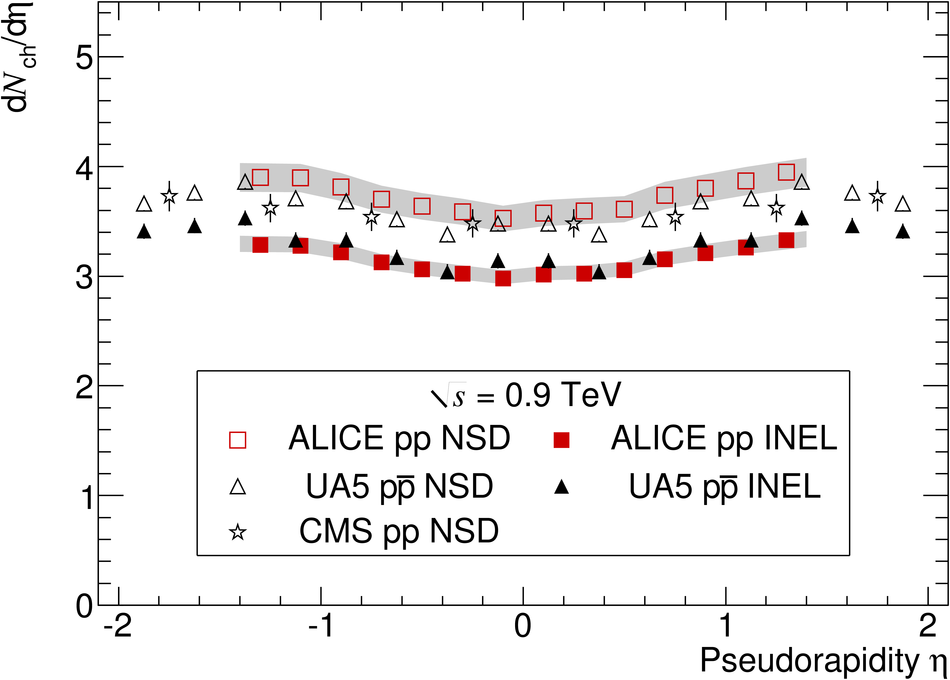## Figure 7

 Charged-particle pseudorapidity density in the central rapidity region in proton--proton and proton--antiproton interactions as a function of the centre-of-mass energy. The dashed and solid lines (for INEL and NSD interactions, respectively) show a fit with a power-law dependence on energy. Note that data points at the same energy have been slightly shifted horizontally for visibility.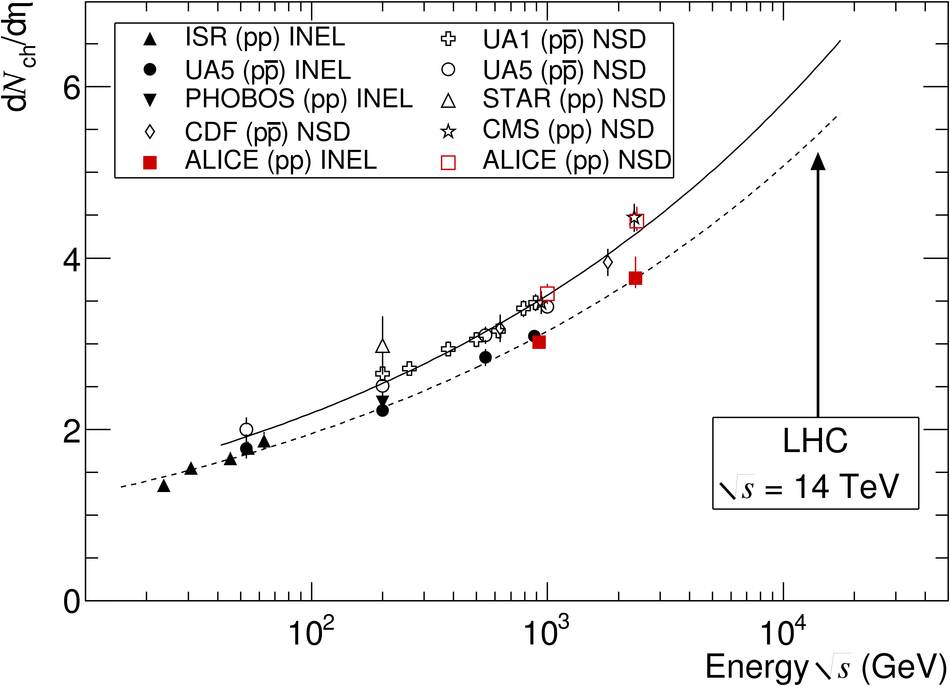## Figure 8

 Corrected multiplicity distributions in three pseudorapidity ranges for NSD events. The solid lines show NBD fits. Error bars represent statistical uncertainties and shaded area systematic ones. Top: Data at $\sqrt{s} = 0.9~$TeV. The ALICE measurement for |$\eta$| < 0.5 is compared to the UA5 data at the same energy \cite{ua5_mult3}. In the inset the ratio of these two measurements is shown, the shaded area represents our combined statistical and systematic uncertainty, and the error bars those of UA5. Bottom: Data at $\sqrt{s} = 2.36~$TeV. Note that for |$\eta$| < 01.0 and |$\eta$| < 01.3 the distributions have been scaled for clarity by the factor indicated.## Figure 9

 Expanded views of the low-multiplicity region of corrected multiplicity distributions for INEL and NSD events, top for 0.9 TeV and bottom for 2.36 TeV data. The gray bands indicate the systematic uncertainty. Distribution for NSD events are not normalized to unity but scaled down in such a way that the distributions for INEL and NSD events match at high multiplicities, which makes the difference at low multiplicity clearly visible. Left: data at $\sqrt{s} = 0.9~$TeV. Right: data at $\sqrt{s} = 2.36~$TeV. Note that for |$\eta$| < 1.0 and |$\eta$| < 1.3 the distributions have been scaled for clarity by the factor indicated.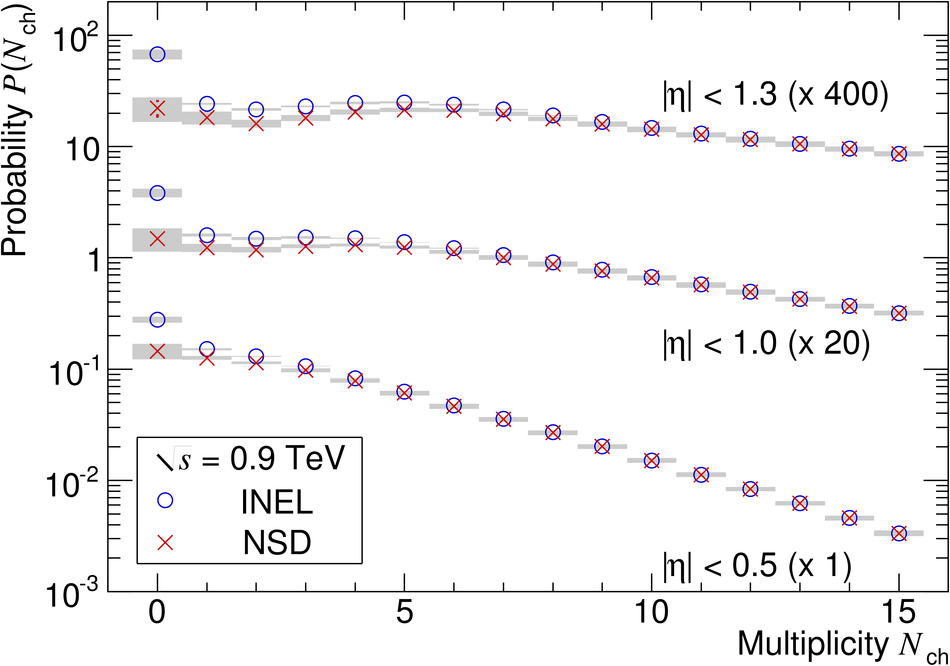## Figure 10

 Comparison of measured multiplicity distributions for INEL events to models for the pseudorapidity range |$\eta$| < 1.0.Predictions are shown based on the PHOJET model  (solid line) and PYTHIA tunes: D6T  (dashed line), ATLAS CSC  (dotted line), and Perugia-0 ] (dash-dotted line).The error bars for data points represent statistical uncertainties, the shaded areas represent systematic uncertainties. Left: data at 0.9 TeV. Right: data at 2.36 TeV. For both cases the ratios between the measured values and model calculations are shown in the lower part with the same convention. The shaded areas represent the combined statistical and systematic uncertainties.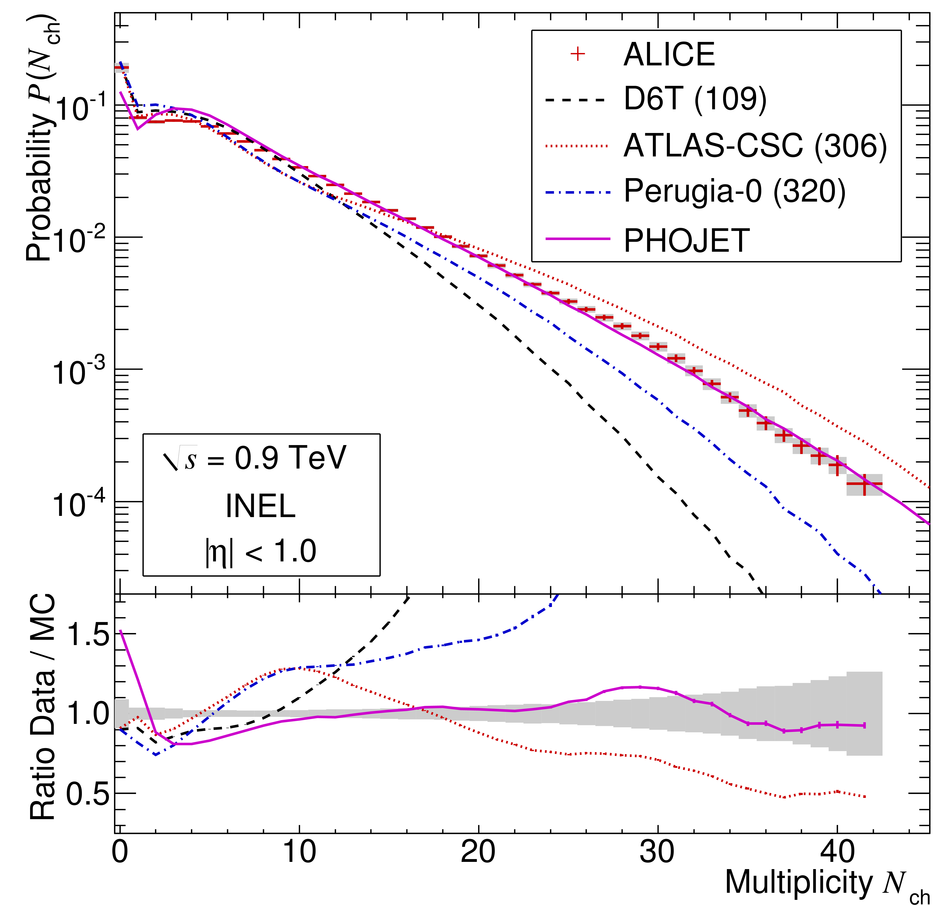## Figure 11

 Energy dependence of the $C_q$-moments () of the multiplicity distributions measured by UA5  and ALICE at both energies for NSD events in two different pseudorapidity intervals. The error bars represent the combined statistical and systematic uncertainties. The data at 0.9 TeV are displaced horizontally for visibility.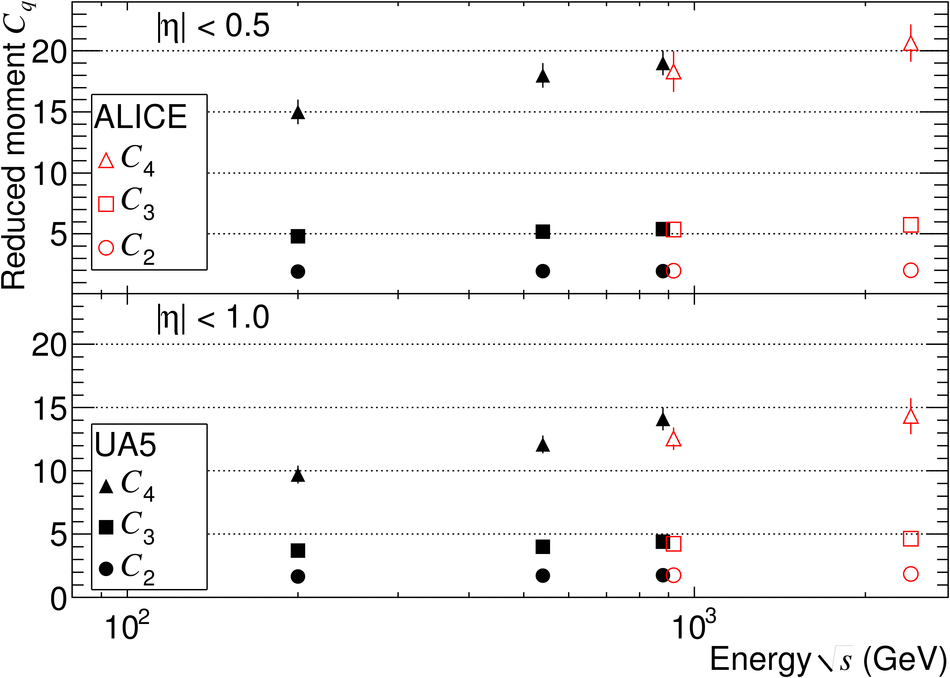## Figure 12

 Comparison of multiplicity distributions in KNO variables measured by UA5 [18,19] in proton-antiproton collisions at $\sqrt{s} = 0.2~$TeV and $0.9~$TeV, and by ALICE at $\sqrt{s} = 0.9~$TeV and $2.36~$TeV, for NSD events in |$\eta$| < 0.5. In the lower part the ratio between ALICE measurements at 0.9 TeV and 2.36 TeV is shown. The error bars represent the combined statistical and systematic uncertainties.